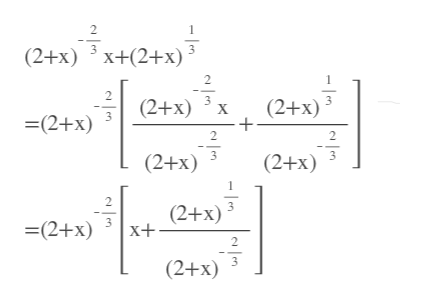# The question says "factor the expression":(2+x)-2/3x+(2+x)1/3 I factored out the power of (2+x) with the smallest exponent, which is (2+x)-2/3 , but the next step (this is an example from a text chapter) says  (2+x)-2/3(x+(2+x)), and I'm not sure how to get to the first x in the parentheses.

Question
1 views

The question says "factor the expression":

(2+x)-2/3x+(2+x)1/3

I factored out the power of (2+x) with the smallest exponent, which is (2+x)-2/3 , but the next step (this is an example from a text chapter) says

(2+x)-2/3(x+(2+x)), and I'm not sure how to get to the first x in the parentheses.

check_circle

Step 1

They got the first x from the first term. Th...help_outlineImage Transcriptionclose2 (2+x) 3 X+(2+x) 2 2 (2+x) 3x (2+x) 3 =(2+x) 2 2 (2+x) (2+x) 2 (2+x) 3 X+ =(2+x) 2 (2+x) fullscreen

### Want to see the full answer?

See Solution

#### Want to see this answer and more?

Solutions are written by subject experts who are available 24/7. Questions are typically answered within 1 hour.*

See Solution
*Response times may vary by subject and question.
Tagged in

### Other